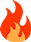Find the correct result . Remember the maths i...

#Find the correct result . Remember the maths is exact

692°
8,572
8222
50

Find the correct result . Remember the maths is exact 👌

1+4=5
2+5=12
3+6=21
5+8=____.

26 Dimers
47
501
6

45

1
591
3

45

72
324
9

45

Deal Major
6,915
23041
280

High school maths

111
350
3

45

Deal Subedar
0
1347
11

High school maths

Asking reasoning question and saying maths problem!

Deal Subedar
195
2915
40

45

Deal Major
6,915
23041
280
Expand
Time_pass wrote:

Asking reasoning question and saying maths problem!

It is maths
Without maths..you can’t solve this ..so yeah it is maths!
You don’t need anything else other than maths..so yeah this is maths!

Deal Subedar
0
1347
11
Expand

It is maths
Without maths..you can’t solve this ..so yeah it is maths!
You don’t need anything else other than maths..so yeah this is maths!

4*1 + 1
5*2 + 2
6*3 + 3
8*5 + 5
Yeah depends on the level of abstraction!
At the bottom layer everything is Maths😛

128
253
3

High school maths

@bobby_123
Are you trying for fun with this reasoning que.DD has many member to solve complex problem, so Post something challengingDeal Major
6,915
23041
280
Expand
Time_pass wrote:

4*1 + 1
5*2 + 2
6*3 + 3
8*5 + 5
Yeah depends on the level of abstraction!
At the bottom layer everything is Maths😛

It is..
Else there is no need for questions as such (more complex) in mathematics Olympiad (high school days)
Probably just a sub section under it!

Deal Captain
8,572
8222
50
Expand
Dev-m wrote:

@bobby_123
Are you trying for fun with this reasoning que.DD has many member to solve complex problem, so Post something challengingOk .. wait for a minute

Entertainer
452
6773
83

Mere hisaab se to 34 bhi ho sakta hai…128
253
3
Expand
bobby_123 wrote:

Ok .. wait for a minute

Mr. Robot is alone enough for any que. @getreadyBlogger
277
5477
86
vijkap201 wrote:

Mere hisaab se to 34 hota hai…That was my answer in 8th standard. My school used to have some kind of NTSE preparation tests at school level. The coach always used to insist that questions might look simple but they are not and most of the questions demand out of box thinking.

Whoever answered that question had the answer as 45, but mine 34. My first solution to the problem was 45 too and I thought why would they ask this question if it was so obvious. I went to out of the box thinking mode and came at the conclusion that the obvious answer was a trap (out of the box thinking was to add everything).

He had picked out my paper and asked me to come to the board to explain it. I did and after that he stopped insisting too much on out of box thinkingPS- I didn’t take NTSE exams in 10th because our school trained us for 2 years but in 10th our Principal insisted that it would be a bad idea to do anything else but 10th exam.

Entertainer
452
6773
83
Expand
rsai01 wrote:

That was my answer in 8th standard. My school used to have some kind of NTSE preparation tests at school level. The coach always used to insist that questions might look simple but they are not and most of the questions demand out of box thinking.

Whoever answered that question had the answer as 45, but mine 34. My first solution to the problem was 45 too and I thought why would they ask this question if it was so obvious. I went to out of the box thinking mode and came at the conclusion that the obvious answer was a trap (out of the box thinking was to add everything).

He had picked out my paper and asked me to come to the board to explain it. I did and after that he stopped insisting too much on out of box thinking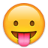PS- I didn’t take NTSE exams in 10th because our school trained us for 2 years but in 10th our Principal insisted that it would be a bad idea to do anything else but 10th exam.

Aapka final answer kya hai???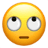1+4 = 5

we have to find the correct result

Expand
Dev-m wrote:

@bobby_123
Are you trying for fun with this reasoning que.DD has many member to solve complex problem, so Post something challenging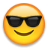Read question once again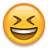Blogger
277
5477
86
Expand
vijkap201 wrote:

Aapka final answer kya hai???I can’t be wrong for the second after the entire class laughed at my out of box thinking of 34. My coach had told that I can’t be making up my own random rules even though it seems logical.

I will keep it simple at 45Entertainer
452
6773
83
Rosh_0007 wrote:

1+4 = 5

we have to find the correct result

First series is crystal clear… Problem starts with second onwards…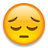Deal Major
6,915
23041
280
Rosh_0007 wrote:

1+4 = 5

we have to find the correct result

haha

Deal Major
6,915
23041
280
Expand
vijkap201 wrote:

First series is crystal clear… Problem starts with second onwards…No..he mean to say
1+4=5 is the only right one among all the 4 statementsBlogger
277
5477
86
Expand
vijkap201 wrote:

First series is crystal clear… Problem starts with second onwards…That is what, you can’t make own rules even though it seems logical. From the second line on you can’t assume that the value of second line addition has to be added to answer from first, because you didn’t do anything like that to the first line to derive that answer. First line holds the key to the logic of this question.

Entertainer
452
6773
83
Expand
rsai01 wrote:

I can’t be wrong for the second after the entire class laughed at my out of box thinking of 34. My coach had told that I can’t be making up my own random rules even though it seems logical.

I will keep it simple at 45In an excel sheet, cumulative total is found this way… Ain’t it???suspended
0
229
1

45 bachcho wale question mat puch…thoda level bdha apna hutiye

Deal Major
6,915
23041
280

Found this on stackexchange..incase if anyone is wondering about the possible outcomes1 + 4 = 5
2 + 5 = 12
3 + 6 = 21
5 + 8 = ?

1 + 4 = 5
2 + 5 = 2 + 2(5) = 12
3 + 6 = 3 + 3(6) = 21
5 + 8 = 5 + 5(8) = 45
ALGORITHM: A + A(B) = C

1 + 4 = 1 + 4 + (0) = 5
2 + 5 = 2 + 5 + (5) = 12
3 + 6 = 3 + 6 + (12) = 21
5 + 8 = 5 + 8 + (21) = 34
ALGORITHM: A + B + C’ = C, where C’ is the previous answer
ANS = 34

1 + 4 = 5 = 5
2 + 5 = (5 + 2) + (5) = 12
3 + 6 = (7 + 2) + (12) = 21
5 + 8 = (9 + 2) + (21) = 32
ALGORITHM: for {X=5, C = X + C’ , X = X+2 };, where C’ is the previous answer. A and B not used in equation,
ANS = 32

1 + 4 = 5
2 + 5 = 7 (base 5) =12
3 + 6 = 9 (base 4) = 21
5 + 8 = 13 (base 3) = 111
ALGORITHM: for {X=6, C = (A + B)^(10 → X), X -1} (First answer in Base6, then Base 5, then 4, etc…)
ANS = 111

1 + 4 = 5
2 + 5 = 7 (base 5) =12
3 + 6 = 9 (base 4) = 21
4 + 7 = 11 (base 3) = 102
5 + 8 = 13 (base 2) (aka binary) = 1101
ALGORITHM: for {X=6, C = (A + B)^(10 → X), X -1} (First answer in Base6, then
Base 5, then 4, etc…including “missing” numbers
ANS = 1101

Expand
Time_pass wrote:

Asking reasoning question and saying maths problem!

School kya band hue, OP started taking classes hereEntertainer
452
6773
83

Hats off… I could think of first two solutions..Entertainer
452
6773
83

Hats off… I could think of first two solutions..